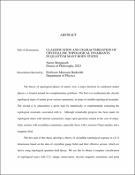## CLASSIFICATION AND CHARACTERIZATION OF CRYSTALLINE TOPOLOGICAL INVARIANTS IN QUANTUM MANY-BODY STATESThe theory of topological phases of matter, now a major direction in condensed matter physics, is framed around two complementary problems. The first is to mathematically classify topological states of matter given various symmetries, in terms of suitable topological invariants. The second is to characterize a given state by numerically or experimentally extracting the topological invariants associated with it. Although remarkable progress has been made for topological states with internal symmetries, major open questions remain in the case of many-body systems with crystalline symmetries, especially those with a nonzero Chern number and a magnetic field. The first part of this thesis develops a theory of crystalline topological response in (2+1) dimensions based on the idea of crystalline gauge fields and their effective actions, which we derive using topological quantum field theory. We use this to obtain a complete classification of topological states with $\U(1)$ charge conservation, discrete magnetic translation, and point group rotation symmetries, finding several new invariants. We separately consider symmetry-enriched topological states of bosons, which admit anyonic excitations with fractional statistics, and invertible fermionic states, which do not. The second part of this thesis focusses on numerically extracting these invariants from many-body invertible states. First we study two quantized invariants, the discrete shift, and a charge polarization which is quantized by rotational symmetries. We show how to extract these invariants in multiple different ways, which include the fractional charge bound to lattice defects, as well as the angular and linear momentum of magnetic flux. Thereafter, we obtain a \textit{complete} characterization of the theoretically predicted invariants, by studying the expectation value of the ground state under partial rotation operators. An immediate application of these ideas is to fully characterize the celebrated Hofstadter model of spinless free fermions on a square lattice. Although the Chern number and filling were first computed in this model in 1982, our theory predicts seven nontrivial invariants, including four new invariants which depend on the crystalline symmetry. We compute these numerically and obtain several additional colorings of Hofstadter's butterfly.